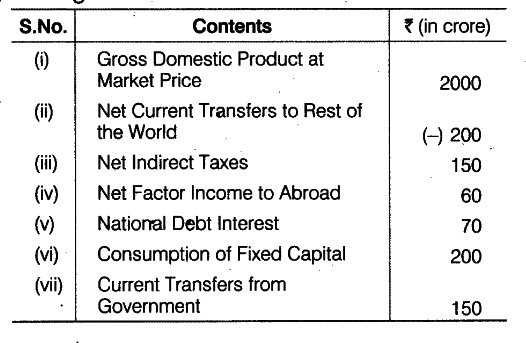# Calculate Net National Disposable Income from the following data:

Calculate Net National Disposable Income from the following data:ND\${{P}_{Fc}}\$ = GDPMP - Consumption of Fixed Capital - Net Indirect Taxes = 2000 - 200 -150 = Rs 1650 crore Net National Disposable Income = Net Domestic Income (NDPFC) + Net Indirect Tax - Net Factor Income to Abroad + Net Current Transfers from Rest of the World
= Rs [1650 + (300-100)-150+250] lakh = Rs 1950 lakh